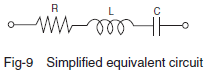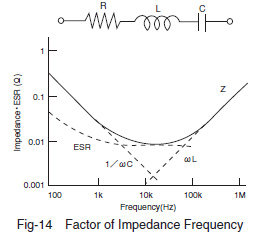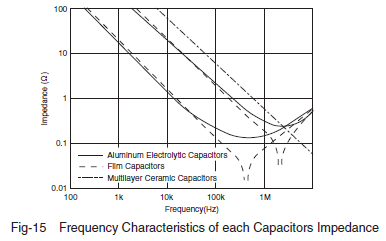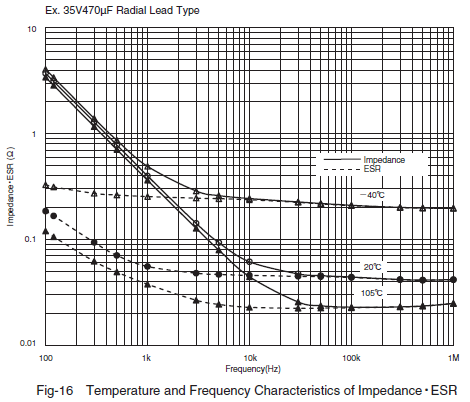### Aluminum electrolytic capacitor Frequency Characteristics of Impedance

When a capacitor is applied with a voltage with the frequency changed, the impedance (Z), a factor of preventing the AC current changes as shown in (Fig. 14). This is the impedance-frequency characteristics of the capacitor.
(Fig. 9) is a simplified model of an equivalent circuit of an aluminum electrolyte capacitor. (Fig. 14) shows dotted lines representing a breakdown of the impedance-frequency characteristic curve into components (C, R and L). As can be seen in this figure, the impedance-frequency characteristics are a composition of C, R and L frequency characteristics.The value 1/ωC shows the pure capacitive reactance graphically presented by a straight line going downward at an angle of 45°, and ωL shows the pure inductive reactance graphically presented by a straight line going upward at 45̊. R shows the equivalent series resistance (ESR). At a range of lower frequencies, the R curve goes downward due to the dielectric loss frequency-dependence. At a range of higher frequencies, the R curve tends to be almost flat since resistance of electrolyte and paper separator is dominant and independent on frequency. Equation (3) shows this tendency.

Because the impedance characteristics of an aluminum electrolyte capacitor depend on resistance of the electrolyte and paper separator, the Z value at the self-resonant frequency tends to be relatively higher, as shown by the solid line in (Fig. 15). The resistance of the electrolyte varies depending on temperature: as the temperature rises, the impedance decreases; and as the temperature decreases, the impedance increases, as shown in (Fig. 16).